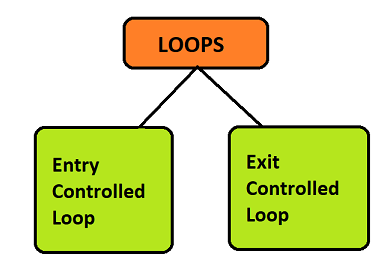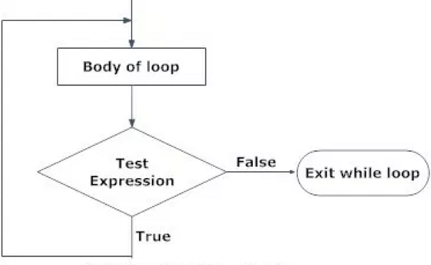×

Search anything:

# do while loop in C

#### C Programming Software Engineering do while loopGet this book -> Problems on Array: For Interviews and Competitive Programming

The iteration statements allow a set of instructions to be performed repeatedly until a certain condition is fulfilled.These are also called as loops or looping statements.These loops save the programmer from writing long lines of codes performing the same task over and over again. C provides three kinds of loops:

• for loop
• while loop
• do-while loop

All loop constructs of C repeat a set of statements as long as a specified condition remains true.This specified condition is generally referred to as a loop control. For all these loop statements , a true condition is any non zero value. A zero value indicates a false condition.

## Parts of a loop

Loops consist of four major elements that govern the exexution and working of a loop:

1. Initialization Expression(s) The initialization of the control variable takes place under this.
2. Test Expression The expression whose truth value decides whether the loop-body will get executed or not.• In an entry-controlled loop the test expression is evaluated before entering into a loop.
• In an exit-controlled loop the test expression is evaluated before exiting from the loop.
1. Update Expression(s) These change the value of loop variable(s).

2. The Body of the loop The statements that are executed repeatedly form the body of the loop.

The do-while loop executes at least once even when the test-expression is false initially.

Syntax:

``````do{
statement;
}
while(test-expression);
``````

Order of execution:1. First we enter the loop and print/execute the statements once.
2. Then the test expression is checked
3. If it evaluates to true, the body of the loop is executed repeatedly, till the test expression evalutes to false
4. If it evaluates to false , the loop is exited.

Example:

``````#include <stdio.h>
int main()
{
int i=0;
do{
printf("Hello\n");
}while(i==1);
printf("Out of loop");
}
``````

Output:

``````Hello
Out of loop
``````

### `while` loop vs `do while` loop

1. Syntax
``````while(condition){
//statements;
}
``````
``````do{
//statements;
}while(condition);
``````
1. loop type

While is an entry controlled loop whereas do-while is an exit controlled loop.

1. Iterations

In while loop do not occur if the condition is false whereas in do-while occur atleast once even if the condition is false.

1. Example

while loop

``````    int i=0,sum=0;
while(i<=10){
sum = sum + i;
i++;
}
``````

do-while loop

``````    int i=0, sum=0;
do{
sum = sum + i;
i++; }
while(i<=10);
``````

### Application

• Used whenever we want the statement inside the loop to be executed atleast once.
• Useful if you want the code to output a menu to a screen so that the menu is guaranteed to show atleast once.

## Question

#### do-while loop is an entry or exit controlled loop?

exit
entry
both
none
Since the test expression is checked at the end of the loop, therefore it is an exit controlled loop. In case of while loop the condition is checked before entering the loop, and hence it is an entry controlled loop.